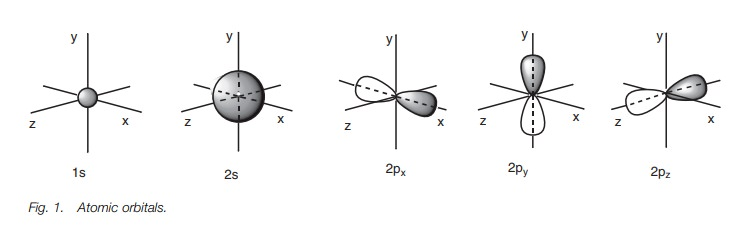Home | | Organic Chemistry | Atomic structure of carbon

# Atomic structure of carbonThe atomic orbitals available for the six electrons of carbon are the s orbital in the first shell, the s orbital in the second shell and the three p orbitals in the second shell.

ATOMIC STRUCTURE OF CARBON

## Atomic orbitals

The atomic orbitals available for the six electrons of carbon are the s orbital in the first shell, the s orbital in the second shell and the three p orbitals in the second shell. The 1s and 2s orbitals are spherical in shape. The 2p orbitals are dumbbell in shape and can be assigned 2px, 2py or 2pz depend-ing on the axis along which they are aligned.

## Energy levels

The 1s orbital has a lower energy than the 2s orbital which has a lower energy than the 2p orbitals. The 2p orbitals have equal energy (i.e. they are degenerate).

## Electronic configuration

Carbon is in the second row of the periodic table and has six electrons which will fill up lower energy atomic orbitals before entering higher energy orbitals (aufbau principle). Each orbital is allowed a maximum of two elec-trons of opposite spin (Pauli exclusion principle). When orbitals of equal energy are available, electrons will occupy separate orbitals before pairing up (Hund’s rule). Thus, the electronic configuration of a carbon atom is 1s2 2s2 2px1 2py1.

## Atomic orbitals

Carbon has six electrons and is in row 2 of the periodic table. This means that there are two shells of atomic orbitals available for these electrons. The first shell closest to the nucleus has a single s orbital – the 1s orbital. The second shell has a single s orbital (the 2s orbital) and three p orbitals (3x2p). Therefore, there are a total of five atomic orbitals into which these six electrons can fit. The s orbitals are spherical in shape with the 2s orbital being much larger then the 1s orbital. The p orbitals are dumbbell-shaped and are aligned along the x, y and z axes. Therefore, they are assigned the 2px, 2py and 2pz atomic orbitals (Fig. 1).## Energy levels

The atomic orbitals described above are not of equal energy (Fig. 2). The 1s orbital has the lowest energy. The 2s orbital is next in energy and the 2p orbitals have the highest energies. The three 2p orbitals have the same energy, meaning that they are degenerate.## Electronic configuration

Carbon is in the second row of the periodic table and has six electrons which will fill up the lower energy atomic orbitals first. This is known as the aufbau princi-ple. The 1sorbital is filled up before the 2sorbital, which is filled up before the 2p orbitals. The Pauli exclusion principle states that each orbital is allowed a maxi- mum of two electrons and that these electrons must have opposite spins. There- fore, the first four electrons fill up the 1s and 2s orbitals.  The electrons in each orbital have opposite spins and this is represented in Fig. 3 by drawing the arrows pointing up or down. There are two electrons left to fit into the remaining 2p orbitals. These go into separate orbitals such that there are two half-filled orbitals and one empty orbital. Whenever there are orbitals of equal energy, electrons will only start to pair up once all the degenerate orbitals are half filled. This is known as Hund’s rule.The electronic configuration for carbon is 1s2 2s2 2px1 2py1. The numbers in superscript refer to the numbers of electrons in each orbital. The letters refer to the types of atomic orbital involved and the numbers in front refer to which shell the orbital belongs.

Study Material, Lecturing Notes, Assignment, Reference, Wiki description explanation, brief detail
Organic Chemistry: Structure and bonding : Atomic structure of carbon |